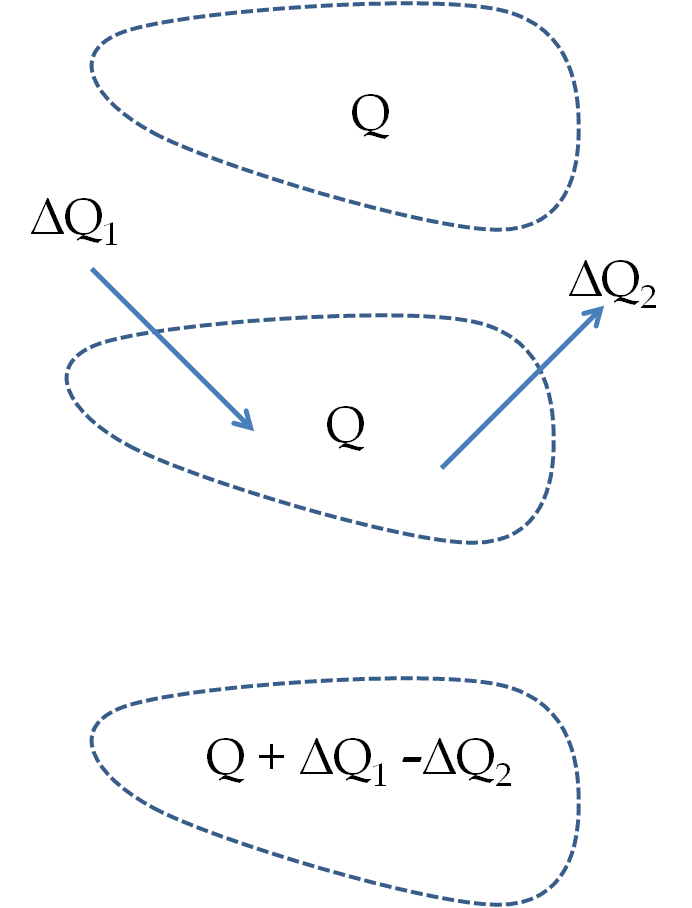## Section28.3Principle of Conservation of Charge

A fundamental property of nature is that the electric charge is conserved in every process. That is, the net charge of the universe does not change with time.

If you look at the charges $\Delta Q_1$ and $\Delta Q_2$ that enter or leave any region of space in some interval $\Delta t\text{,}$ then you will find that the charge in the region at the end of the interval will be equal to the charge $Q$ at the beginning plus charges that entered minus charges that left the region in that time.

\begin{equation*} |\text{Net change}| = |\text{Amount entered} - \text{Amount exited}|. \end{equation*}Creation or destruction of net non-zero charge has not been seen in any experiment. Whenever charges seem to be created in a process, they always occur in pairs of equal and opposite types. For instance, when a neutron with zero net charge decays in the nuclear beta decay of radioactivity, two charged particles, an electron and a proton, are produced along with a neutral particle called neutrino, actually anti-electron neutrino. Thus, the net charge after the reaction is zero as at the beginning.

\begin{align*} \amp \textrm{Reaction: }\ n\ \ \rightarrow\ \ \ e \ \ +\ \ p\ \ +\ \ \bar\nu_e, \\ \amp \textrm{Charges: }\ (0)\ \rightarrow\ (-e)\ \ \ (+e) \ \ \ \ (0). \end{align*}

Similarly, in electrochemistry, equal and opposite charges appear at the two electrodes so that the charges separate by equal amount leaving the solution neutral if it was neutral at the start.